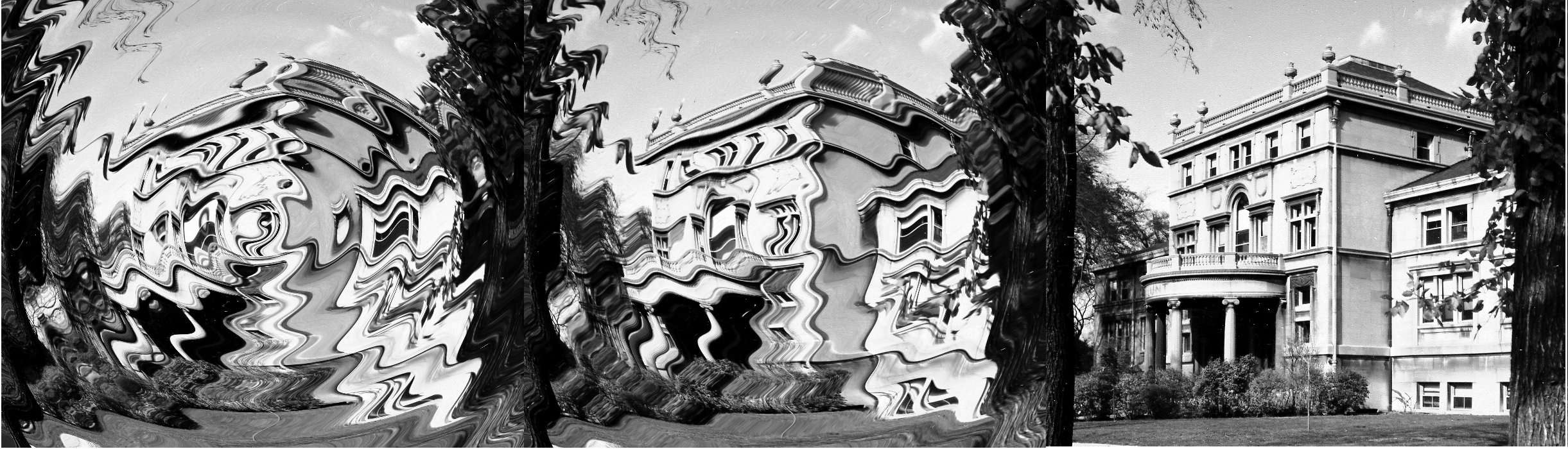May Midwestern Microlocal Meeting
Northwestern University, Evanston, IL

May 16th and 17th, 2015

Schedule

Talks took place in 105 Lunt Hall and were open to the mathematical public.

Saturday
9:30Breakfast
10:00Maciej Zworski, UC Berkeley
Scattering resonances as viscosity limits
11:00Yaiza Canzani, Harvard University and IAS
Pointwise Weyl law and random waves
2:00Richard Melrose, MIT
Nodal degeneration for the Laplacian and the Weil-Petersson metric
3:00Long Jin, UC Berkeley
A local trace formula for Anosov flows
High frequency estimates for the Helmholtz equation and applications to boundary integral equations
5:00Wine and cheese in Lunt common room
Sunday
9:30Breakfast
10:00Peter Hintz, Stanford University
Quasilinear wave equations and microlocal analysis with rough coefficients
11:00Wilhelm Schlag, University of Chicago
On long-term dynamics of nonlinear wave equations
1:00Lunch at Jared's

Abstracts

High frequency estimates for the Helmholtz equation and applications to boundary integral equations

I will discuss joint work with Euan Spence and Jared Wunsch on high frequency estimates for the Helmholtz equation in exterior domains as well as in interior domains subject to impedance boundary conditions. The motivation for these estimates comes from numerical analysis.

• Yaiza Canzani

Pointwise Weyl law and random waves

There are several questions about Laplace eigenfunctions that have proved to be extremely hard to deal with and remain unsolved. Among these are the study of the asymptotic behavior of the Hausdorff measure of the zero set, the number of critical points and the number of nodal domains. A natural approach is to randomize the problem and ask all the previous questions for random linear combinations of eigenfunctions. It turns out that when doing so one needs to control with some accuracy the kernel of the spectral projection operator onto high frequency windows. In this talk I will explain how to get good off diagonal estimates for the kernel of the projection near non self-focal points.

• Peter Hintz

Quasilinear wave equations and microlocal analysis with rough coefficients

We present a global approach to the study of nonlinear wave equations, based on microlocal analysis on compactified spaces with boundary. Specifically, we consider quasilinear waves, where the metric depends on the solution; analyzing the corresponding wave operator requires a robust framework for proving microlocal regularity results for operators with rough coefficients, in the spirit of work by Beals and Reed, and we will describe the key ingredients of this framework. Joint work with András Vasy.

• Long Jin

A local trace formula for Anosov flows

In this talk, we present a local trace formula for Anosov flows on compact manifolds which relates Pollicott-Ruelle resonances to the periods of closed orbits. As an application, we show a weak lower bound for the counting function for resonances in a strip and thus the infinitude of the resonances.

• Richard Melrose

Nodal degeneration for the Laplacian and the Weil-Petersson metric

I will describe joint work with Xuwen Zhu in which we analyze the behaviour of the Laplacian on the fibres of a (multi-)Lefschetz fibration. We use this to describe the behaviour of the constant curvature metric on a Riemann surface undergoing nodal degeneration and applying this to the universal case of moduli space deduce the asymptotics of the Weil-Petersson metric, so extending recent work of Mazzeo and Swoboda.

• Wilhelm Schlag

On long-term dynamics of nonlinear wave equations

We will review some of the developments in the asymptotic theory of semilinear wave equations of the focusing type. These equations admit soliton solutions and we address the description of the dynamical picture after long time. Ideas from both PDE and dynamics will be used.

• Maciej Zworski

Scattering resonances as viscosity limits

In practically all situations resonances can be defined as limits of L2 eigenvalues of operators which regularize the Hamiltonian at infinity. For instance, Pollicott--Ruelle resonances in the theory of dynamical systems are given by viscosity limits: adding a Laplacian to the generator of an Anosov flow gives an operator with a discrete spectrum; letting the coupling constant go to zero turns eigenvalues into the resonances (joint work with S Dyatlov). This principle seems to apply in all other settings where resonances can be defined and I will explain it in the simplest case of scattering by compactly supported potentials. The method has also been numerically investigated in the chemistry literature as an alternative to complex scaling.

Organizers

Kiril Datchev (Purdue), Antônio Sá Barreto (Purdue), and Jared Wunsch (Northwestern).

Please email any questions to kdatchev@purdue.edu.